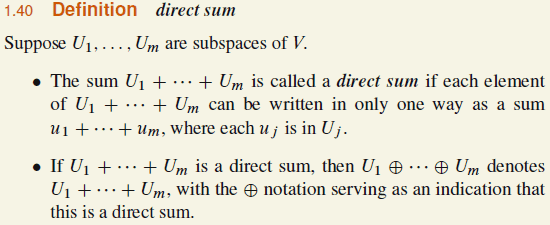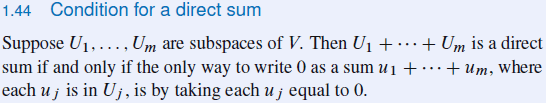# Confusion about the Direct Sum of Subspaces

• I
Calculuser
In "Sheldon Axler's Linear Algebra Done Right, 3rd edition", on page 21 "internal direct sum", or direct sum as the author uses, is defined as such:Following that there is a statement, titled "Condition for a direct sum" on page 23, that specifies the condition for a sum of subspaces to be internal direct sum. In proof the author proves the uniqueness of this condition as far as I get, which is understandable, but I do not think that proves the statement itself:Question 1: How can we prove that checking if the only way to write ##0## vector as the sum of ##u_1+u_2+...+u_m## where each ##u_i\in U_i## is taking each ##u_i## equal to ##0##? is sufficient for a sum of subspaces to be internal direct sum?

Question 2: Is it possible for some of those subspaces ##U_1, U_2, . . . , U_m## have some elements in common other than ##0## vector while their summation still produces an internal direct sum? If not, why?

Mentor
2022 Award
In "Sheldon Axler's Linear Algebra Done Right, 3rd edition", on page 21 "internal direct sum", or direct sum as the author uses, is defined as such:

View attachment 244008

Following that there is a statement, titled "Condition for a direct sum" on page 23, that specifies the condition for a sum of subspaces to be internal direct sum. In proof the author proves the uniqueness of this condition as far as I get, which is understandable, but I do not think that proves the statement itself:

View attachment 244010

Question 1: How can we prove that checking if the only way to write ##0## vector as the sum of ##u_1+u_2+...+u_m## where each ##u_i\in U_i## is taking each ##u_i## equal to ##0##? is sufficient for a sum of subspaces to be internal direct sum?
Indirect. Assume a vector ##u\neq 0## has two expressions and show that ##0## has two expressions, too, in that case.
In the other direction is trivial: if each vector can be expressed uniquely, then especially the zero vector.
Question 2: Is it possible for some of those subspaces ##U_1, U_2, . . . , U_m## have some elements in common other than ##0## vector while their summation still produces an internal direct sum? If not, why?
It's not possible. Why? See your proof of the answer to question 1.

Calculuser
Indirect. Assume a vector ##u\neq 0## has two expressions and show that ##0## has two expressions, too, in that case.
In the other direction is trivial: if each vector can be expressed uniquely, then especially the zero vector.

What we want to infer from statements some other statement by some inference rule is what happens in proofs as I try to detail as much as possible through my basic knowledge on propositional calculus.

If every element of ##U_1+U_2+...+U_m## can be uniquely written as a sum of ##u_1+u_2+...+u_m## where each ##u_i## is in ##U_i##, then ##U_1+U_2+...+U_m## is a direct sum. So, $$P\Longrightarrow Q \qquad $$ where, $$P: \text{every element of } U_1+U_2+...+U_m \text{ can be uniquely written as a sum of } u_1+u_2+...+u_m \text{ where each } u_i \text{ is in } U_i$$ $$Q: U_1+U_2+...+U_m \text{ is a direct sum}$$
By modus ponens we would like to get ##Q##, $$P\Longrightarrow Q, P \vdash Q \qquad $$
##P\Longrightarrow Q## is by definition true so that all we have to do is to derive ##P## statement as a sufficient condition to check. Hence, after testing ##P## is true we can infer ##Q##.

If we take the negation of statement ##P##, $$\lnot P: \text{ there exists some elements of } U_1+U_2+...+U_m \text{ that cannot be written uniquely as a sum of } u_1+u_2+...+u_m \text{ where each } u_i \text{ is in } U_i$$
Hence if we take ##v## from ##U_1+U_2+...+U_m##, which can be written in two different sums, $$v=u_1+u_2+...u_m, \text{ } u_i\in U_i \qquad $$ $$v=w_1+w_2+...+w_m, \text{ } w_i\in U_i \qquad $$
Subtracting #### from ####, $$0=(v_1-w_1)+(v_2-w_2)+...(v_m-w_m), \text{ } u_i, w_i \in U_i \qquad $$
We can interpret ####, #### and #### as such: if we take some arbitrary vector ##v## other than ##0## (##v\neq 0##), we can use it to write ##0## vector from #### and #### as another sum shown in #### in addition to ##0=0+0+...+0##, which is always the case because ##0## is in every ##U_i## by definition of subspace; and the summation of ##0## where each ##0## taking from ##U_i## guarantees ##0## in ##U_1+U_2+...+U_m## by such a sum ##0=0+0+...+0##. In other words, we can always generate another sum of zero vector out of any vector different from zero vector can be written in different ways. Therefore we can phrase: if any nonzero vector can be written by different sums, then zero vector can be written by different sums. Contrapositively, this means if zero vector cannot be written by different sums, then any nonzero vector cannot be written by different sums. Representing this statement as, $$R_1\Longrightarrow R_2 \qquad $$
where, $$R_1: \text{ any nonzero vector can be written by different sums}$$ $$R_2: \text{ zero vector can be written by different sums}$$
Thinking of ##R_1\Longrightarrow R_2## as a categorical proposition where ##R_1## is a set whose elements having property of nonzero vectors ##v## that can be written by at least two different sums; and ##R_2## is a set whose elements having property of zero vector that can be written by at least two different sums. In Venn diagram representation, $$R_1 \setminus R_2 = \emptyset$$
and the complement set these two ##R_1## and ##R_2## (##(R_1 \cup R_2)'##) represents the set of all vectors ##v##, ##0## included, having the property of a unique representation as a sum of ##u_1+u_2+...+u_m##. Therefore if there exists an element in ##R_2##, we can say not direct sum; or if not, then a direct sum.

Do these all make sense or not? Did I miss any point, skip any logical step, or such in all these?

Mentor
2022 Award
I don't understand what you are doing here. ##P \Longleftrightarrow Q## is the definition 1.40 of a direct sum as far as I can see what's in your pictures, so there is no work to do. What's left is to show, that already the zero vector does it.

Now as every vector can be uniquely written as sum of ##u_j##, this is also true for ##u=0##.

This leaves us with the task: If ##0_U=\sum_j 0_{U_j}## is the only way to write ##0_U \in U##, then there is only one way to write any other vector ##v \in U##, which you did ... somewhere hidden in the waterfall of letters.

You are correct to assume two sums for ##v## as in  and , and also to build the difference  (up to the typo that it should read ##u_j-w_j##). Now you only need two arguments:
1. The ##U_j## are subspaces, so ##u_j-w_j \in U_j##.
2. The zero vector has only one representation, namely ##0_U=\sum_j 0_{U_j}##.
Therefore ##u_j-w_j = 0_{U_j}## for all ##j##. End of proof.

You do not need all these things you have written. Just ##,,## and the two arguments above. I assume this is somewhere in what you wrote, but I have difficulties to find it.